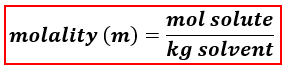# Problem: What is the molality of a 32.0% by weight aqueous solution of acetone (C  3H6O)?A. 8.10B. 7.80C. 7.12D. 6.56E. 5.74

###### FREE Expert Solution

We’re being asked to calculate for the molality of 32.0 % by weight aqueous solution of acetone (C3H6O).

When calculating for molality, we use the following equation:Aqueous solution → water is the solvent

Solute → acetone

We will calculate the molality of the solution using the following steps:

Step 1. Determine the composition of the solution.
Step 2. Calculate the moles of the solute.
Step 3. Calculate the mass of the solvent (in kg).
Step 4. Calculate the molality of the solution.

Step 1. Determine the composition of the solution.###### Problem Details

What is the molality of a 32.0% by weight aqueous solution of acetone (C  3H6O)?

A. 8.10

B. 7.80

C. 7.12

D. 6.56

E. 5.74

What scientific concept do you need to know in order to solve this problem?

Our tutors have indicated that to solve this problem you will need to apply the Molality concept. You can view video lessons to learn Molality. Or if you need more Molality practice, you can also practice Molality practice problems.

What is the difficulty of this problem?

Our tutors rated the difficulty ofWhat is the molality of a 32.0% by weight aqueous solution o...as medium difficulty.

How long does this problem take to solve?

Our expert Chemistry tutor, Dasha took 4 minutes and 56 seconds to solve this problem. You can follow their steps in the video explanation above.

What professor is this problem relevant for?

Based on our data, we think this problem is relevant for Professor Costanza's class at USF.